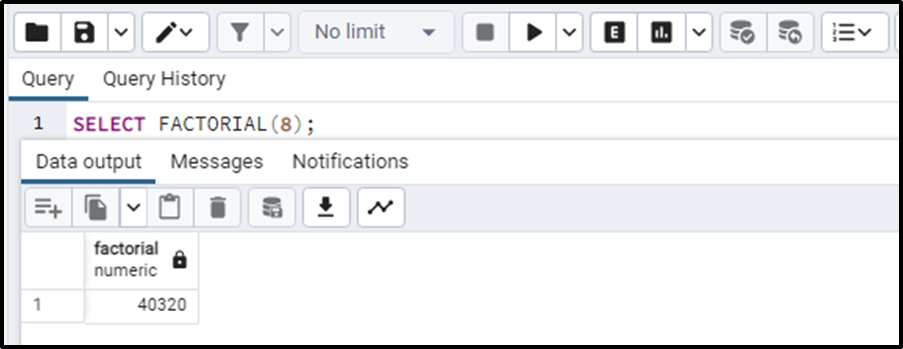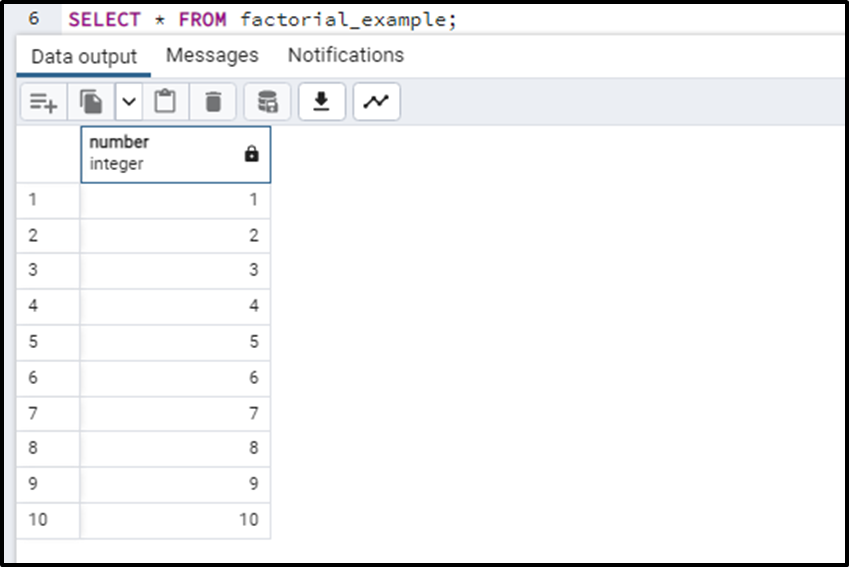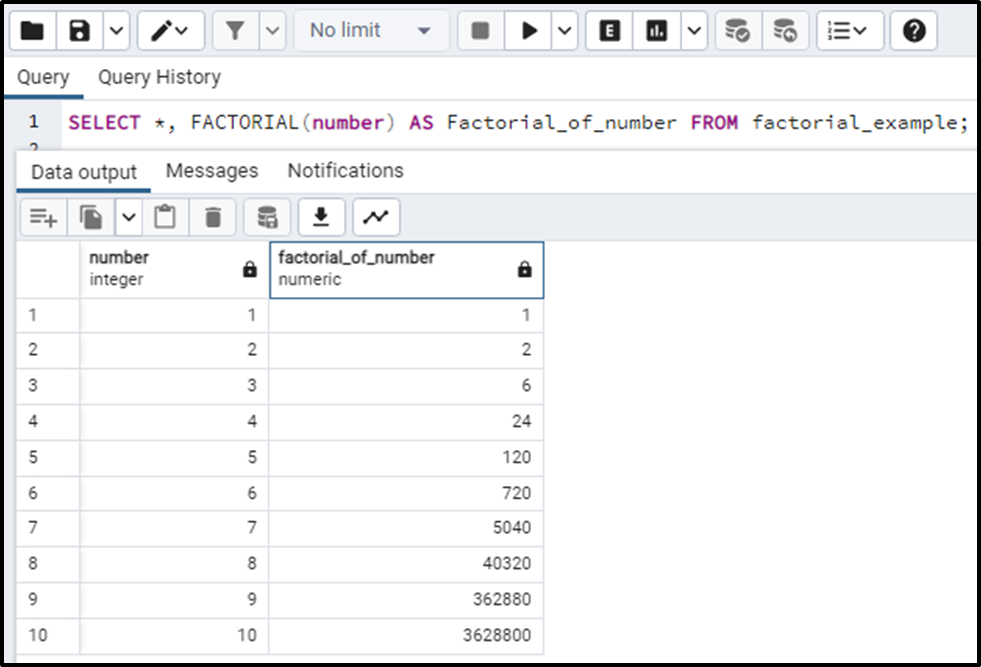##### How to Find Factorial of a Number in PostgreSQL

In Postgres, the factorial of a number can be found by using a built-in function that is FACTORIAL(). A number is passed into the function, the FACTORIAL() function will return the factorial of that number. In the previous versions of PostgreSQL, the factorial can also be found using the “!” and “!!” operators. But these operators are now not supported in PostgreSQL 14 and onwards. Let’s find out the method to find the factorial of a number in PostgreSQL.

## How to Find Factorial of a Number in PostgreSQL

To find the factorial of the number in PostgreSQL, we use the FACTORIAL() function that takes a number as an input and returns the value of its factorial. Here is a simple query where we are returning the factorial value of 8:

`SELECT FACTORIAL(8);`

The output of the query will give the factorial value of 8 which is 40320.Let's see how it works with a table column. Firstly, we will create a table using the CREATE TABLE command and then we will insert some values in it. The query for this task will be:

```CREATE TABLE factorial_example(number int);
INSERT INTO factorial_example(number)
VALUES(1),(2),(3),(4),(5),(6),(7),(8),(9),(10);```

This query will create the table and insert values in it. This will result in the following table:Now let’s write the query for finding the factorial and insert the returned value of the FACTORIAL() function in another column. The query will be:

`SELECT *, FACTORIAL(number) AS Factorial_of_number FROM factorial_example;`

We have passed a column named “number” into the FACTORIAL() function. The query will return the table with an extended row of respective factorials of the numbers:So, we can see a column “factorial_of_number” returning the factorials. This is the method to find the factorial of any number in PostgreSQL.

### Conclusion

To find the factorial of a number in Postgresql, the FACTORIAL() function is used. This function takes the number as a parameter and returns the value of its factorial. Before Postgres 14, some operators like ”!” and “!!” were also used but these operators are now not supported by current versions of Postgres.